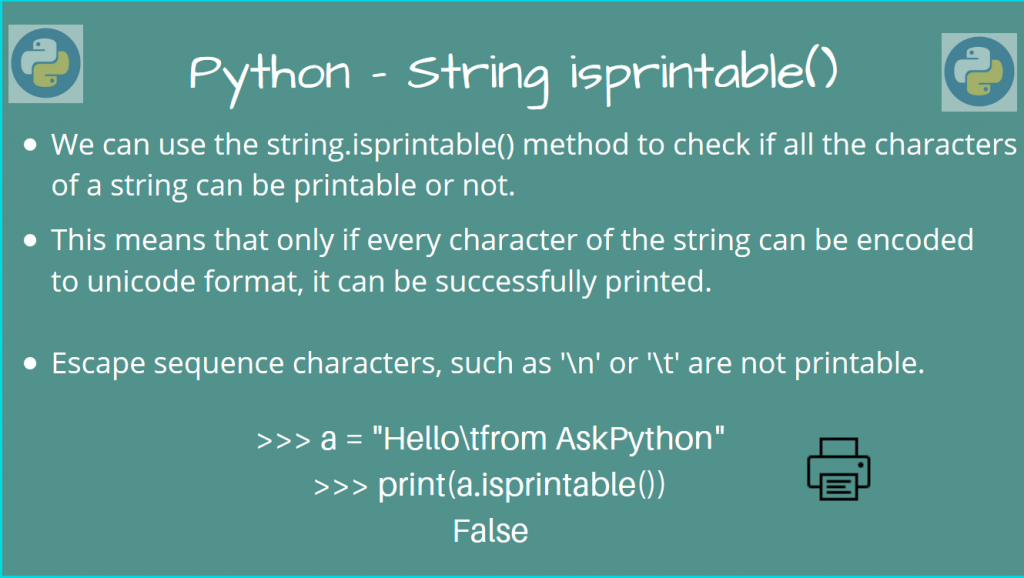# Python String isprintable()The Python String isprintable() function can be used to check if a Python string is printable or not.

What does this mean exactly? Let’s find out!

## Python String isprintable()

Certain characters in Python strings cannot be directly printed to the console (file). They are classified as “Other” or “Separator” in the Unicode Character Database.

Note, the ASCII space character (0x20) is an exception to this, as it can obviously be printed.

Another way of thinking about printable strings is that it can be successfully decoded to a Unicode character. Any non-printable string will throw an error in that regard.

It is roughly equivalent to the following method:

```def isprintable(s, codec='utf8'):
try: s.decode(codec)
except UnicodeDecodeError: return False
else: return True
```

If we can get the decoded string in `utf-8` format, then it can be printed. Otherwise, we simply return `False`.

So let’s check how we can use this function, using some examples.

To use this function, we must call Python string isprintable() method on a string object.

```ret = string.isprintable()
```

`ret` is a Boolean, which is `True`, if the string can be printed. Otherwise, it is `False`.

```>>> a = "Hello from AskPython"
>>> print(a.isprintable())
True
```

Here, all the characters in our string are printable, so it returns `True`.

An empty string is also printable.

```>>> b = ""
>>> print(b.isprintable())
True
```

We cannot print escaped characters like `\n` or `\t`, so if our string has any of the escape sequences, `isprintable()` will return `False`.

```>>> c = "Hello from AskPython\n"
>>> print(c.isprintable())
False

>>> print(d.isprintable())
False
```

There are some characters (like `\u0066` -> f), which can be printed, but others (like `\u0009` -> \t) cannot.

Basically, they must map to a valid unicode character.

```>>> e = "Hello \u0066rom AskPython"
>>> print(e.isprintable())
True
>>> print(e)

>>> print(f.isprintable())
False
>>> print(f)
```

To conclude with the working of the isprintable() method, let’s look at finding out all the non-printable characters in our unicode database.

## Find out all non-printable Characters

The total number of unicode characters is `2^16`, so we will make a loop which goes through every character, and checks if it can be printed or not.

```count = 0

for ascii_val in range(2 ** 16):
ch = chr(ascii_val)
if not ch.isprintable():
count += 1

print(f"Total Number of Non-Printable Unicode Characters = {count}")
```

Output

```Total Number of Non-Printable Unicode Characters = 10249
```

## Conclusion

In this article, we learned how we could use the Python String isprintable() method to check if the characters of a string can be printed or not.